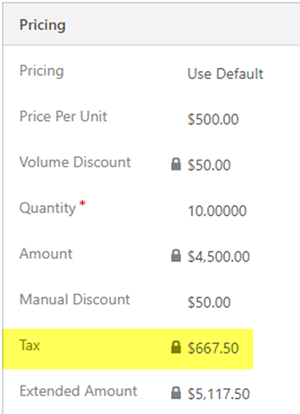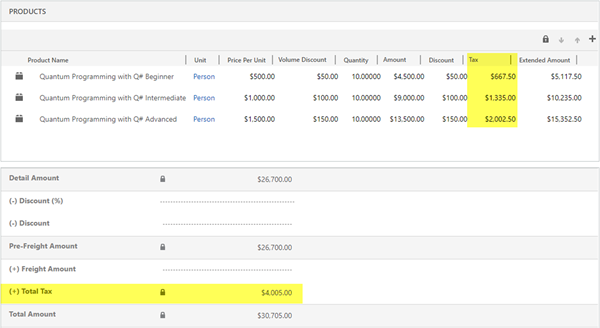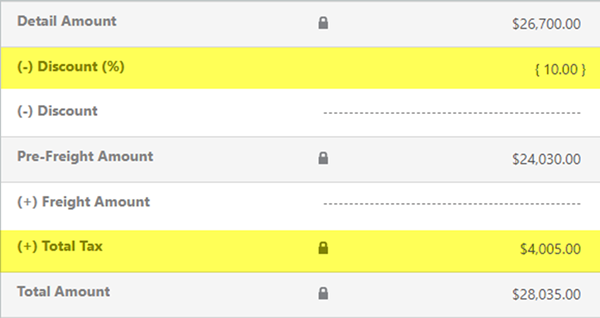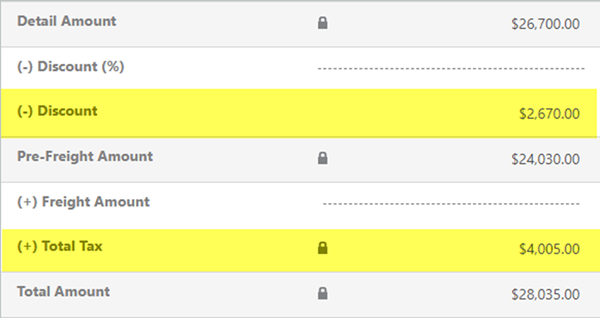# Tax and Discounts Quotes/Orders in Dynamics 365 - Part 1 of 3

### Colin Maitland, 05 September 2018

In the first of this three-part series I will briefly describe how the Tax Amount field for Quote/Order Products and Total Tax Amount field for Quotes/Orders in Microsoft Dynamics 365 work, especially when a Discount has also been applied to Quote/Order.

### TAX AMOUNT AND MANUAL DISCOUNT ON QUOTE/ORDER PRODUCTS

Quote/Order Products have a Tax Amount field that may be used to record the Tax Amount, if any, that is applicable to the Quote/Order Product. This provides a mechanism for adding Tax Amount at the Quote/Order Product level and if required for using different Tax Percentages for different Products; e.g. 15% for all Products except Gift Vouchers which may have a 0% Rate Percentage.

The following image shows an Order Product with a Tax Amount of \$667.50; i.e. (Amount - Manual Discount) * Tax Percentage; e.g. (\$4,500 - \$50) * 15% = \$667.50. In this example there is also a Volume Discount of \$50 per Unit, but this has already been applied prior to the system calculating the Amount.The Extended Amount for the Quote/Order Product is also system calculated; i.e. (Amount - Manual Discount) + Tax Amount; e.g. (\$4,500 - \$50) + \$667.50 = \$5,117.50.

The Price Per Unit, derived from the corresponding Price List Item in the Product Catalogue, therefore needs to be Tax Exclusive, if the Tax Amount field for Quote/Order Products is used, to ensure that the Extended Amount is the correct Tax Inclusive amount.

In Microsoft Dynamics 365 the Tax Amount field on Quote/Order Products is not system calculated. However custom programming may be added to calculate the applicable Tax Amount, if any, for each Quote/Order Product as required.

### TOTAL TAX AND DISCOUNT ON QUOTE/ORDER PRODUCTS

Quotes/Orders have a system calculated Total Tax field.

The following image shows the system calculated Total Tax that has been added to a Quote/Order. This is the sum of the Tax Amount from the related Quote/Order Products; e.g. \$4,005.00.

The Total Amount is also system calculated; i.e. Total Amount = ((Detail Amount - Discount Percent) - Discount Amount) + Freight Amount + Total Tax; e.g. \$30,705.00.It is important to know that the application of a discount at the Quote/Order level does not cause the Total Tax to be adjusted in any way.

The following image shows the unchanged Total Tax after the application of a 10% discount to the Quote/Order:The following image shows the unchanged Total Tax after the application of a \$2,670 discount to the Quote/Order:### CONCLUSION

This review shows that the behaviour of the Total Tax field at Quote/Order Product compared to Tax field at the Quote/Order level is different.

At the Quote/Order Product level the Tax should take into consideration any Manual Discount that has been applied to the Quote/Order Product. This is implied by the fact that the system calculated Extended Amount = (Amount - Manual Discount Amount) + Tax Amount. Any custom programming used to calculate the Tax Amount should calculate the Tax Amount from the (Amount - Manual Discount).

At the Quote/Order level the Total Tax is system calculated and does not take into consideration any Discount, i.e. Discount Percent or Discount Amount, that has been applied to the Quote/Order.

This means that the Discount, if any, applied at the Quote/Order level results in the Detail Amount being discounted but does not include any corresponding adjustment to the Tax Amounts on the related Quote/Order Products; e.g. The Tax Total on the Quote/Order before and after the application of a Discount remains unchanged.

If the Quote/Order Tax Exclusive Total is \$26,700.00 then the Total Tax should be \$4,005.00, assuming a Tax Rate of 15%. However, when a 10% Discount is applied the Quote/Order the Tax Exclusive Total is now \$24,030.00 but the Total Tax remains \$4,005.00, i.e. 16.67% instead of 15% of the discounted Tax Exclusive Total, rather than being adjusted to \$3,604.50.

For some scenarios this will be problematic. In the next two articles I will describe two customisation approaches may be used to solve this.